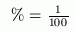﻿ Changing Decimals to Percents
CHANGING DECIMALS TO PERCENTS
by Dr. Carol JVF Burns (website creator)
Follow along with the highlighted text while you listen!

One use for decimals is in working with percents, which are commonplace in everyday life:

• the dress was on sale for $\,40\%\,$ off the original price;
• housing costs rose $\,5\%\,$ last year;
• there was a $\,150\%\,$ increase in telephone activity after the newspaper advertisement.

There are $\,100\,$ cents in a dollar.
A century is $\,100\,$ years.
The word  percent  means  per one hundred .

The symbol  $\%$  is used for percent.
Whenever you see the symbol   $\%$ , you can trade it in for a factor of $\,\frac{1}{100}\,$.
Whenever you see a factor of $\,\frac{1}{100}\,$, it can be traded in for a  $\%$  symbol.
This simple idea is the key to success with percents:Indeed, the symbol  $\%$  even looks a bit like the fraction $\,\frac{1}{100}\,$;
it has the two zeros and the division bar!

To change from a decimal to a percent,
move the decimal point two places to the right and insert the percent symbol.

EXAMPLES:
$0.03 = 3\%$

$2.5 = 250\%$

$1.008 = 100.8\%$

Here's the idea that makes this work:

$\displaystyle 0.03 = \frac{3}{100} = 3\cdot\frac{1}{100} = 3\%$

Notice that the $\displaystyle\,\frac{1}{100}\,$ gets traded in for the percent symbol.

Master the ideas from this section

When you're done practicing, move on to:
Changing Percents to Decimals

Here, you will practice renaming decimals as percents.
For example, $\,0.54\,$ gets renamed as $\,54\%\,$.

Change to a percent: